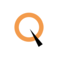Number Pattern Programs in Java

# Number Pattern Programs in Java

4 mins read273 Views 1 Comment
clickHere
Updated on Oct 3, 2023 11:33 IST

Pattern programs in Java are a type of problem that use nested loops to produce different patterns of numbers, stars (*), or other characters. In this blog we will dive deeper specifically into number pattern programs in java.

Number pattern programs in Java display a sequence of numbers in certain patterns. These programs are often used as a practical exercise for learning loop control structures in Java (i.e., for loops, while loops, and do-while loops). The patterns could be anything from a simple pyramid shape to more complex combinations and sequences of numbers.

Explore Pattern Programs in C

## Why Are Number Pattern Programs Important?

• Understanding Control Structures: Helps understanding and mastering the use of loops and control structures.
• Problem-Solving Skills: Enhances logical thinking and problem-solving skills.
• Attention to Detail: Improves attention to detail by focusing on precise pattern generation.
• Preparation for Interviews: Commonly used in coding interviews and assessments.Array Programs in Java | Beginner to Expert Level
Array programs in Java traverse from basic single-dimensional arrays to complex multi-dimensional arrays and dynamic arrays using ArrayList. From initializing and accessing array elements, to advanced operations like sorting and...read moreHow to Return an Array in Java
In Java, methods can return arrays to provide multiple data elements of a consistent type in a single response. There are various ways on how to return an array. Today...read moreA Guide to Power Function in Java
Have you ever wondered how mathematical power functions are implemented in programming languages like Java? In Java, the Math.pow() function is a powerful tool used to raise a number to...read more

## Basic Approach to Solve a Number Pattern Program Using an Example

### Step 1: Understand the Pattern

• Visualize the Pattern: Observe the pattern to understand its structure.
• Identify the Number of Rows and Columns: Determine the number of rows and columns in the pattern.
• Analyze the Sequence: Understand how the numbers are arranged in each row and column.

```   1
212
32123
4321234
```

### Step 2: Initialize the Program

Start the Class and Main Method: Initialize your Java program with a class and a main method.

` `
`public class Main {    public static void main(String[] args) {        // Code goes here    }}Copy code`

### Step 3: Determine and Set the Number of Rows

Set the Number of Rows: Determine how many rows the pattern will have.

` `
`int n = 4; // The pattern has 4 rowsCopy code`

### Step 4: Construct an Outer Loop for Rows

Create the Outer Loop: Set up an outer loop to iterate through the rows.

` `
`for (int i = 1; i <= n; i++) {    // Inner loops will go here}Copy code`

### Step 5: Construct Inner Loops for Columns

Create Inner Loops for Columns: Utilize inner loops to manage the columns and print the numbers.

` `
`for (int j = n; j >= 1; j--) {    if (j <= i) {        System.out.print(j);    } else {        System.out.print(" ");    }}for (int k = 2; k <= i; k++) {    System.out.print(k);}Copy code`

### Step 6: Combine and Execute the Code

Combine All Code Segments: Combine all parts and run the program to print the pattern.

` `
`public class Main {    public static void main(String[] args) {        int n = 4;        for (int i = 1; i <= n; i++) {            for (int j = n; j >= 1; j--) {                if (j <= i) {                    System.out.print(j);                } else {                    System.out.print(" ");                }            }            for (int k = 2; k <= i; k++) {                System.out.print(k);            }            System.out.println();        }    }}Copy code`

Following the above steps, you can systematically approach and solve any number pattern program in Java.

## Top 10 Number Pattern Programs in Java

1. Right-Angle Triangle Pattern
2. Inverted Right-Angle Triangle Pattern
3. Pyramid Number Pattern
4. Inverted Pyramid Number Pattern
5. Diamond Number Pattern
6. Hollow Diamond Number Pattern
7. Pascal’s Triangle Pattern
8. Floyd’s Triangle Pattern
9. Palindromic Number Pattern
10. Binary Number Pyramid Pattern

### Let’s understand each of these one by one in detail :

Right-Angle Triangle Pattern: A simple pattern where each row contains increasing consecutive numbers.

```1
1 2
1 2 3
1 2 3 4
1 2 3 4 5
```

Code

` `
`public class Main {    public static void main(String[] args) {        for (int i = 1; i <= 5; i++) {            for (int j = 1; j <= i; j++) {                System.out.print(j + " ");            }            System.out.println();        }    }}Copy code`

Inverted Right-Angle Triangle Pattern: Similar to the right-angle triangle pattern but inverted.

```1 2 3 4 5
1 2 3 4
1 2 3
1 2
1
```

Code

` `
`public class Main{    public static void main(String[] args) {        for (int i = 5; i >= 1; i--) {            for (int j = 1; j <= i; j++) {                System.out.print(j + " ");            }            System.out.println();        }    }}Copy code`

Pyramid Number Pattern: A pattern of numbers arranged in the form of a pyramid.

```     1
121
12321
1234321
123454321
```

Code

` `
`public class Main {    public static void main(String[] args) {        int n = 5;        for (int i = 0; i < n; i++) {            for (int j = 0; j < n - i; j++) {                System.out.print(" ");            }            for (int k = 0; k <= i; k++) {                System.out.print(k + 1);            }            for (int l = i - 1; l >= 0; l--) {                System.out.print(l + 1);            }            System.out.println();        }    }}Copy code`

Inverted Pyramid Number Pattern: An inverted version of the pyramid number pattern.

```123456789
1234567
12345
123
1
```

Code

` `
`public class Main {    public static void main(String[] args) {        int n = 5;        for (int i = n; i >= 1; i--) {            for (int j = n; j > i; j--) {                System.out.print(" ");            }            for (int k = 1; k < (i * 2); k++) {                System.out.print(k);            }            System.out.println();        }    }}Copy code`

Diamond Number Pattern: A pattern where numbers are arranged in a diamond shape.

```    1
123
12345
1234567
123456789
1234567
12345
123
1
```

Code

` `
`public class Main {    public static void main(String[] args) {        int n = 5;        for (int i = 1; i <= n; i++) {            for (int j = i; j < n; j++) {                System.out.print(" ");            }            for (int k = 1; k < (i * 2); k++) {                System.out.print(k);            }            System.out.println();        }        for (int i = n - 1; i >= 1; i--) {            for (int j = n; j > i; j--) {                System.out.print(" ");            }            for (int k = 1; k < (i * 2); k++) {                System.out.print(k);            }            System.out.println();        }    }}Copy code`

Hollow Diamond Number Pattern: A diamond-shaped pattern with numbers on the border and hollow inside.

```    1
1 1
1   1
1     1
1       1
1     1
1   1
1 1
1
```

Code

` `
`public class Main {    public static void main(String[] args) {        int n = 5;        for (int i = 1; i <= n; i++) {            for (int j = i; j < n; j++) {                System.out.print(" ");            }            for (int k = 1; k <= (2 * i - 1); k++) {                if (k == 1 || k == (2 * i - 1)) {                    System.out.print(1);                } else {                    System.out.print(" ");                }            }            System.out.println();        }        for (int i = n - 1; i >= 1; i--) {            for (int j = n; j > i; j--) {                System.out.print(" ");            }            for (int k = 1; k <= (2 * i - 1); k++) {                if (k == 1 || k == (2 * i - 1)) {                    System.out.print(1);                } else {                    System.out.print(" ");                }            }            System.out.println();        }    }}Copy code`

Pascal’s Triangle Pattern: A triangular pattern where each number is the sum of the two numbers directly above it.

```     1
1 1
1 2 1
1 3 3 1
1 4 6 4 1
```

Code

` `
`public class Main {    public static void main(String[] args) {        int n = 5;        for (int i = 0; i < n; i++) {            int number = 1;            for (int j = 0; j < n - i; j++) {                System.out.print(" ");            }            for (int k = 0; k <= i; k++) {                System.out.print(number + " ");                number = number * (i - k) / (k + 1);            }            System.out.println();        }    }}Copy code`

Floyd’s Triangle Pattern: A right-angle triangle pattern with consecutive numbers.

```1
2 3
4 5 6
7 8 9 10 ```

Code

` `
`public class Main {    public static void main(String[] args) {        int n = 4, number = 1;        for (int i = 1; i <= n; i++) {            for (int j = 1; j <= i; j++) {                System.out.print(number + " ");                number++;            }            System.out.println();        }    }}Copy code`

Palindromic Number Pattern: A pattern where numbers are the same in a row forwards and backward.

```    1
121
12321
1234321
123454321
```

Code

` `
`public class Main {    public static void main(String[] args) {        int n = 5;        for (int i = 1; i <= n; i++) {            for (int j = i; j < n; j++) {                System.out.print(" ");            }            for (int k = 1; k < i; k++) {                System.out.print(k);            }            for (int l = i; l >= 1; l--) {                System.out.print(l);            }            System.out.println();        }    }}Copy code`

Binary Number Pyramid Pattern: A pyramid pattern of binary numbers (0 and 1).

```    0
0 1
0 1 0
0 1 0 1
0 1 0 1 0
```

Code

` `
`public class Main {    public static void main(String[] args) {        int n = 5;        for (int i = 0; i < n; i++) {            for (int j = n - 1; j > i; j--) {                System.out.print(" ");            }            for (int k = 0; k <= i; k++) {                System.out.print((k % 2) + " ");            }            System.out.println();        }    }}Copy code`

## Conclusion

Thus, number pattern programs in Java are an essential part of foundational programming learning and practice. They not only help in understanding the use and manipulation of loops and control statements, but also enhance logical thinking and problem-solving skills.

clickHere

I'm Esha Gupta, a B.Tech graduate in Computer Science & Engineering, specializing in Front End Web Dev. I've interned at GeeksforGeeks & Coding Minutes, fueling my passion for crafting appealing and functional digit... Read Full Bio

(1)

## Trending Technology CoursesProgramming in Java by NPTEL
NPTEL4.6Java Full Stack
Qspider4.6## Top Picks & New Arrivals